# Recent Activity

## Every 4-connected toroidal graph has a Hamilton cycle ★★

Author(s): Grunbaum; Nash-Williams

Conjecture   Every 4-connected toroidal graph has a Hamilton cycle.

Keywords:

## Acyclic list colouring of planar graphs. ★★★

Author(s): Borodin; Fon-Der-Flasss; Kostochka; Raspaud; Sopena

Conjecture   Every planar graph is acyclically 5-choosable.

Keywords:

## Earth-Moon Problem ★★

Author(s): Ringel

Problem   What is the maximum number of colours needed to colour countries such that no two countries sharing a common border have the same colour in the case where each country consists of one region on earth and one region on the moon ?

Keywords:

## Triangle-packing vs triangle edge-transversal. ★★

Author(s): Tuza

Conjecture   Ifhas at mostedge-disjoint triangles, then there is a set of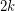edges whose deletion destroys every triangle.

Keywords:

## Odd-cycle transversal in triangle-free graphs ★★

Author(s): Erdos; Faudree; Pach; Spencer

Conjecture   Ifis a simple triangle-free graph, then there is a set of at most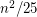edges whose deletion destroys every odd cycle.

Keywords:

## Simultaneous partition of hypergraphs ★★

Author(s): Kühn; Osthus

Problem   Letand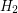be two-uniform hypergraph on the same vertex set. Does there always exist a partition ofintoclasses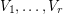such that for both, at least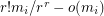hyperedges of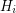meet each of the classes?

Keywords:

## Complexity of the H-factor problem. ★★

Author(s): Kühn; Osthus

An-factor in a graphis a set of vertex-disjoint copies ofcovering all vertices of.

Problem  Letbe a fixed positive real number anda fixed graph. Is it NP-hard to determine whether a graphonvertices and minimum degreecontains and-factor?

Keywords:

## Subgraph of large average degree and large girth. ★★

Author(s): Thomassen

Conjecture   For all positive integers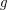and, there exists an integersuch that every graph of average degree at leastcontains a subgraph of average degree at leastand girth greater than.

Keywords:

## Turán number of a finite family. ★★

Author(s): Erdos; Simonovits

Given a finite familyof graphs and an integer, the Turán number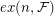ofis the largest integersuch that there exists a graph onvertices withedges which contains no member ofas a subgraph.

Conjecture   For every finite familyof graphs there exists ansuch that.

Keywords:

## Subdivision of a transitive tournament in digraphs with large outdegree. ★★

Conjecture   For allthere is an integer such that every digraph of minimum outdegree at least contains a subdivision of a transitive tournament of order.

Keywords:

## Large induced forest in a planar graph. ★★

Author(s): Abertson; Berman

Conjecture   Every planar graph onverices has an induced forest with at leastvertices.

Keywords:

## Lovász Path Removal Conjecture ★★

Author(s): Lovasz

Conjecture   There is an integer-valued functionsuch that ifis any-connected graph andand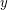are any two vertices of, then there exists an induced pathwith endsandsuch thatis-connected.

Keywords:

## Partition of a cubic 3-connected graphs into paths of length 2. ★★

Author(s): Kelmans

Problem   Does every-connected cubic graph onvertices admit a partition intopaths of length?

Keywords:

## Decomposing an eulerian graph into cycles with no two consecutives edges on a prescribed eulerian tour. ★★

Author(s): Sabidussi

Conjecture   Letbe an eulerian graph of minimum degree, and let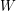be an eulerian tour of. Thenadmits a decomposition into cycles none of which contains two consecutive edges of.

Keywords:

## Decomposing an eulerian graph into cycles. ★★

Author(s): Hajós

Conjecture   Every simple eulerian graph onvertices can be decomposed into at mostcycles.

Keywords:

## Decomposing a connected graph into paths. ★★★

Author(s): Gallai

Conjecture   Every simple connected graph onvertices can be decomposed into at mostpaths.

Keywords:

## Melnikov's valency-variety problem ★

Author(s): Melnikov

Problem   The valency-varietyof a graphis the number of different degrees in. Is the chromatic number of any graphwith at least two vertices greater thanKeywords:

## Do any three longest paths in a connected graph have a vertex in common? ★★

Author(s): Gallai

Conjecture   Do any three longest paths in a connected graph have a vertex in common?

Keywords:

## Coloring the union of degenerate graphs ★★

Author(s): Tarsi

Conjecture   The union of a-degenerate graph (a forest) and a-degenerate graph is-colourable.

Keywords:

## Arc-disjoint strongly connected spanning subdigraphs ★★

Author(s): Bang-Jensen; Yeo

Conjecture   There exists an inetegerso that every-arc-connected digraph contains a pair of arc-disjoint strongly connected spanning subdigraphs?

Keywords: